# 第五门课 序列模型(Sequence Models)

## 第一周 循环序列模型（Recurrent Neural Networks）

### 1.1 为什么选择序列模型？（Why Sequence Models?）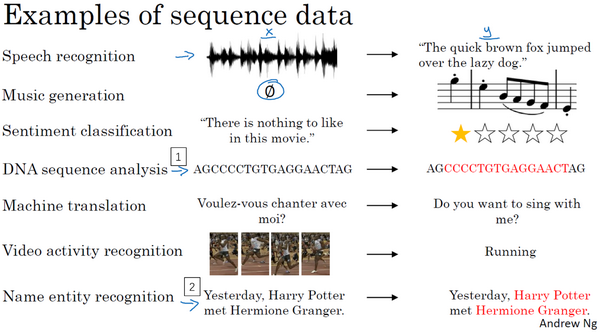###1.2 数学符号（Notation）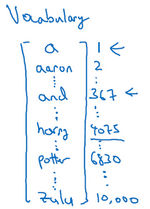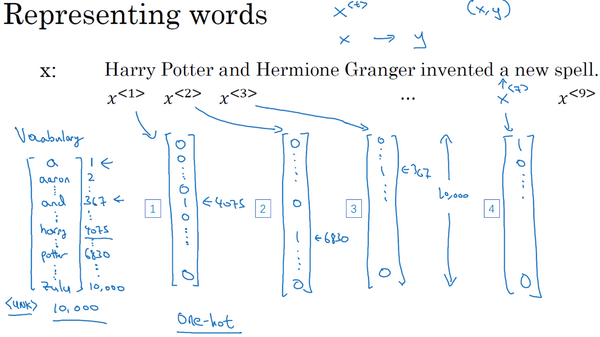and在词典里排第367，所以$x^{<3>}$就是第367行是1，其余值都是0的向量（上图编号3所示）。如果你的词典大小是10,000的话，那么这里的每个向量都是10,000维的。

###1.3 循环神经网络模型（Recurrent Neural Network Model）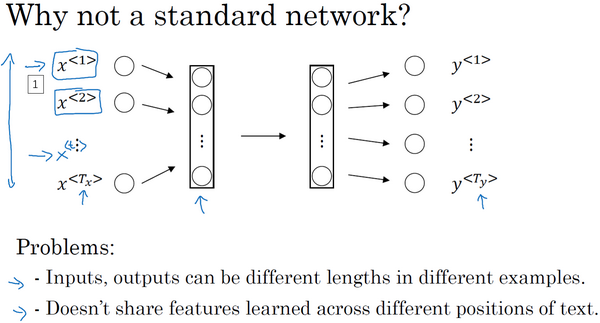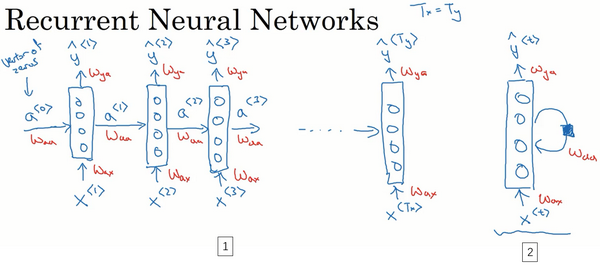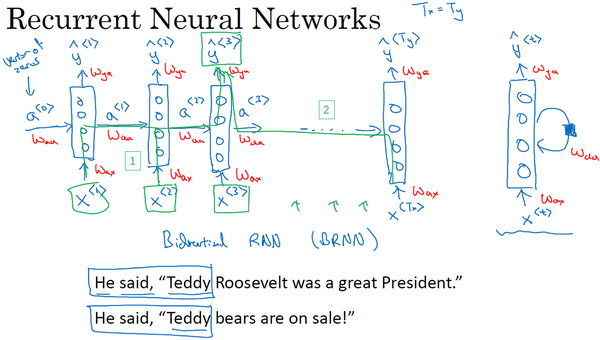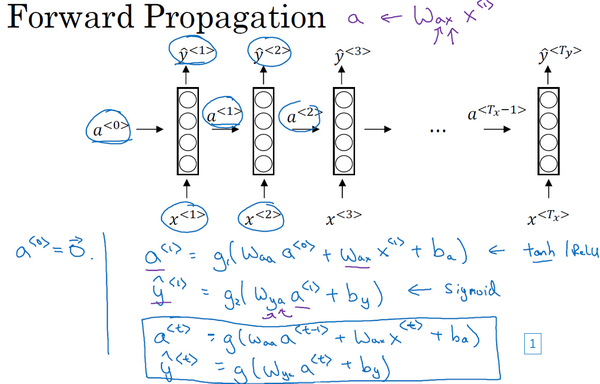$a^{<1>} = g_{1}(W_{{aa}}a^{< 0 >} + W_{{ax}}x^{< 1 >} + b_{a})$

$\hat y^{< 1 >} = g_{2}(W_{{ya}}a^{< 1 >} + b_{y})$

$a^{< t >} = g_{1}(W_{aa}a^{< t - 1 >} + W_{ax}x^{< t >} + b_{a})$

$\hat y^{< t >} = g_{2}(W_{{ya}}a^{< t >} + b_{y})$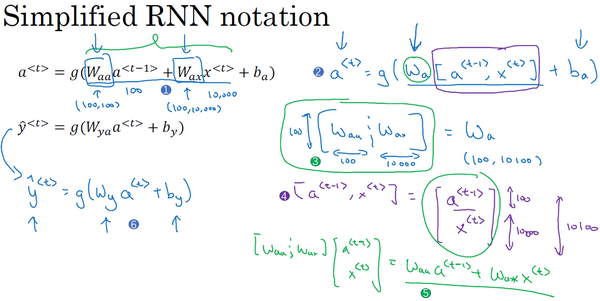RNN前向传播示意图：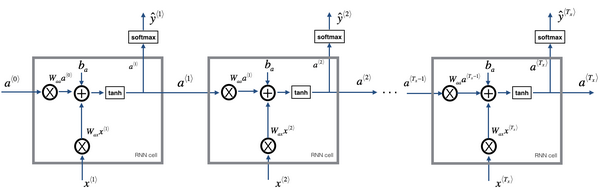###1.4 通过时间的反向传播（Backpropagation through time）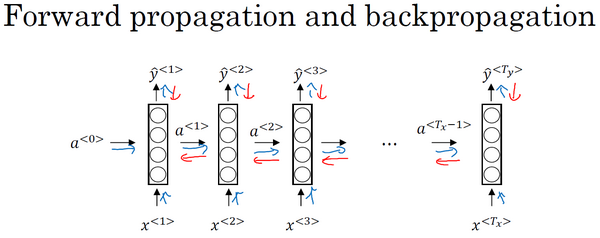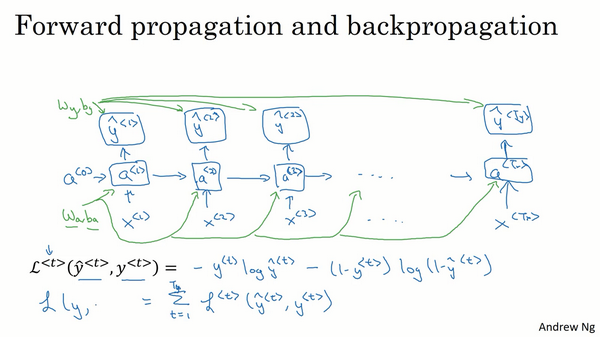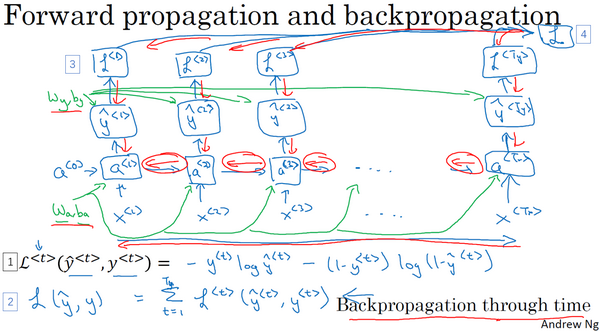$L^{}( \hat y^{},y^{}) = - y^{}\log\hat y^{}-( 1- y^{})log(1-\hat y^{})$

$L(\hat y,y) = \ \sum_{t = 1}^{T_{x}}{L^{< t >}(\hat y^{< t >},y^{< t >})}$

RNN反向传播示意图：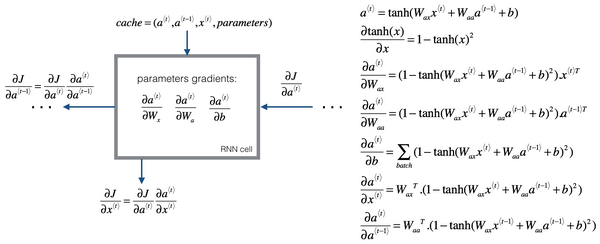###1.5 不同类型的循环神经网络（Different types of RNNs）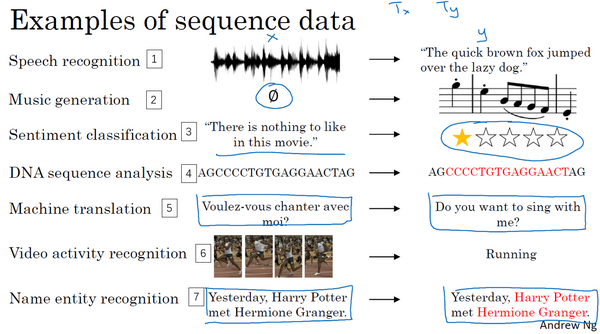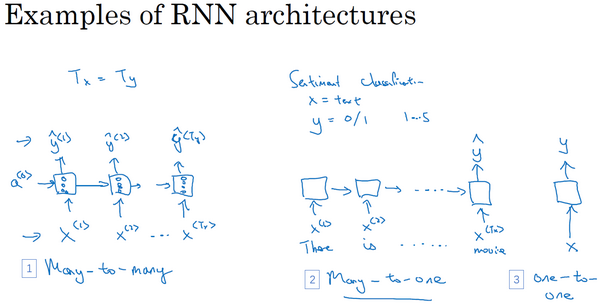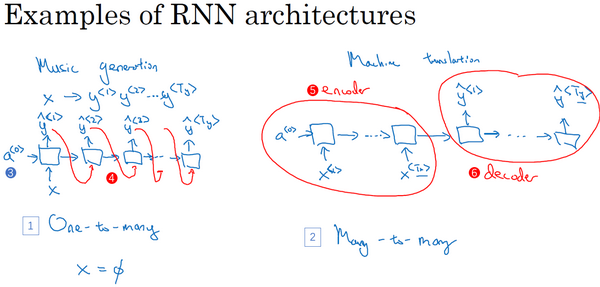###1.6 语言模型和序列生成（Language model and sequence generation）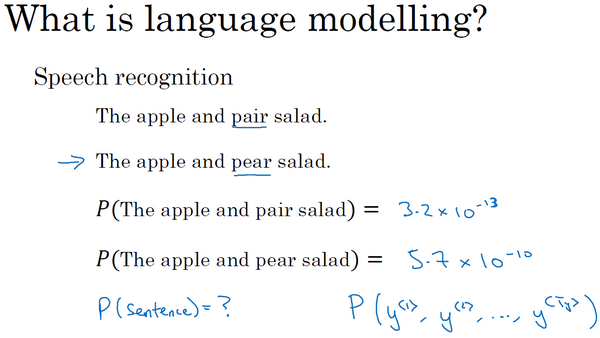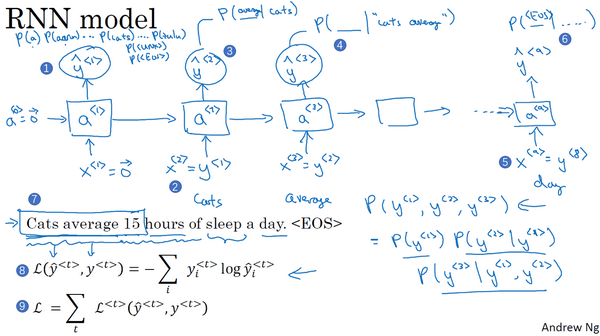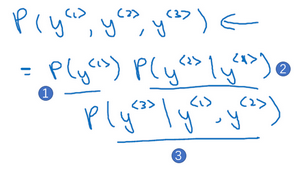###1.7 对新序列采样（Sampling novel sequences）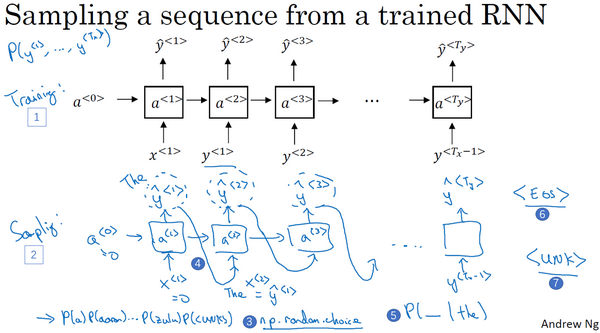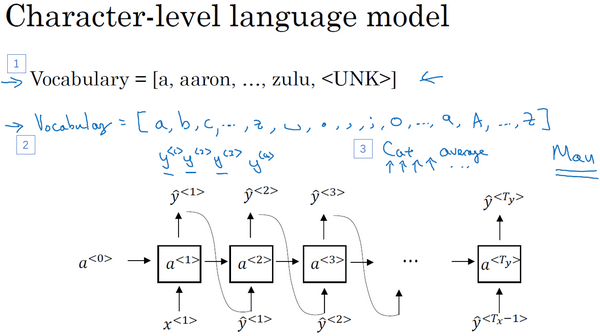The mortal moon hath her eclipse in love.

And subject of this thou art another this fold.

When besser be my love to me see sabl's.

For whose are ruse of mine eyes heaves.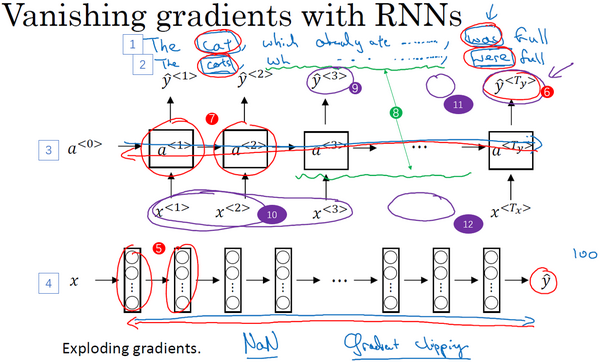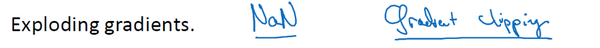###1.9 GRU单元（Gated Recurrent Unit（GRU））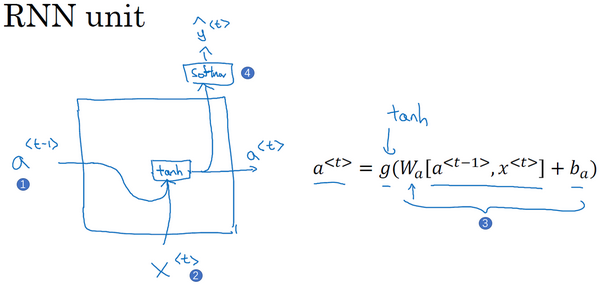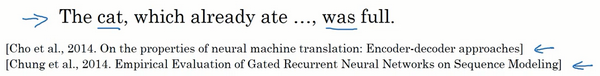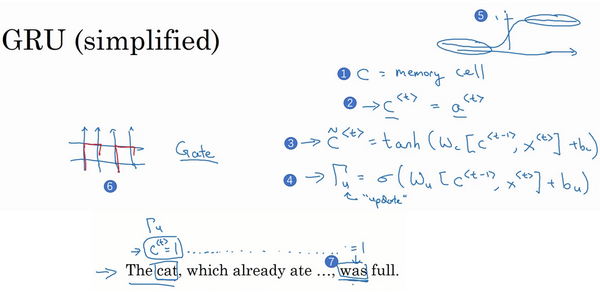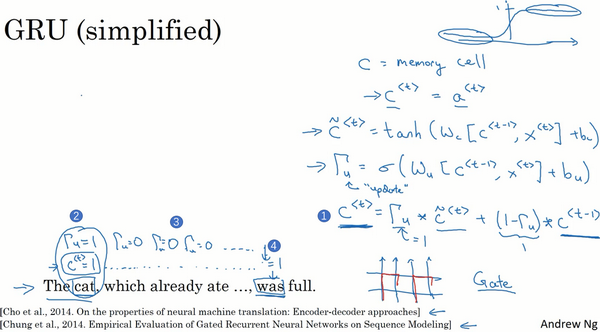GRU单元输入$c^{}$（下图编号1所示），对于上一个时间步，先假设它正好等于$a^{}$，所以把这个作为输入。然后$x^{}$也作为输入（下图编号2所示），然后把这两个用合适权重结合在一起，再用tanh计算，算出${\tilde{c}}^{}$${\tilde{c}}^{} =tanh(W_{c}\left\lbrack c^{},x^{} \right\rbrack +b_{c})$，即$c^{}$的替代值。